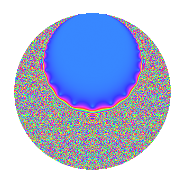# Properties

 Label 3.21.bLevel 3 Weight 21 Character orbit b Rep. character $$\chi_{3}(2,\cdot)$$ Character field $$\Q$$ Dimension 6 Newforms 1 Sturm bound 7 Trace bound 0

# Related objects

## Defining parameters

 Level: $$N$$ = $$3$$ Weight: $$k$$ = $$21$$ Character orbit: $$[\chi]$$ = 3.b (of order $$2$$ and degree $$1$$) Character conductor: $$\operatorname{cond}(\chi)$$ = $$3$$ Character field: $$\Q$$ Newforms: $$1$$ Sturm bound: $$7$$ Trace bound: $$0$$

## Dimensions

The following table gives the dimensions of various subspaces of $$M_{21}(3, [\chi])$$.

Total New Old
Modular forms 8 8 0
Cusp forms 6 6 0
Eisenstein series 2 2 0

## Trace form

 $$6q - 4122q^{3} - 2125200q^{4} - 96401232q^{6} + 559607916q^{7} - 1087483482q^{9} + O(q^{10})$$ $$6q - 4122q^{3} - 2125200q^{4} - 96401232q^{6} + 559607916q^{7} - 1087483482q^{9} - 10880304480q^{10} + 63957886128q^{12} + 17847879276q^{13} - 487764624960q^{15} - 317472843648q^{16} + 9564155313120q^{18} - 14958020195124q^{19} + 25978999888044q^{21} - 71051286019680q^{22} + 294048705803904q^{24} - 397204775017050q^{25} + 1077025592505798q^{27} - 2034284889273504q^{28} + 3061064118304800q^{30} - 2182905165483348q^{31} + 3811197222014400q^{33} - 7566372644651136q^{34} + 6910111676037744q^{36} - 1934341462168404q^{37} - 15813602040849876q^{39} + 44482420967535360q^{40} - 86552595699685920q^{42} + 58194987502184076q^{43} - 81854223046416000q^{45} + 151411391218915776q^{46} - 154489681080035712q^{48} + 188165158287453522q^{49} - 117657755976045312q^{51} - 230547517796956704q^{52} + 489800358305152272q^{54} - 393208608470140800q^{55} + 1211071992434087436q^{57} - 1639827118548168480q^{58} + 2591328282252814080q^{60} - 2999109545381544468q^{61} + 444547436097192876q^{63} - 83569554960393216q^{64} + 120735307117251360q^{66} + 4488155969890198476q^{67} - 3511312424189451648q^{69} - 1812568842584740800q^{70} - 10414532525611288320q^{72} + 15738405804262597836q^{73} - 20871070048457840250q^{75} + 27275280223392575328q^{76} - 13410777930056987040q^{78} + 17679940751103803436q^{79} - 13011625055229011514q^{81} - 35403353112903693120q^{82} + 60397598618988279264q^{84} - 22576574831711316480q^{85} + 17858271599894295360q^{87} - 95000209108729447680q^{88} + 179562325304911301280q^{90} - 6461010485165713512q^{91} - 24838278011742494484q^{93} - 311754310853935072896q^{94} + 184738040137761352704q^{96} + 223240336245548394636q^{97} - 178130031568853028480q^{99} + O(q^{100})$$

## Decomposition of $$S_{21}^{\mathrm{new}}(3, [\chi])$$ into irreducible Hecke orbits

Label Dim. $$A$$ Field CM Traces $q$-expansion
$$a_2$$ $$a_3$$ $$a_5$$ $$a_7$$
3.21.b.a $$6$$ $$7.605$$ $$\mathbb{Q}[x]/(x^{6} + \cdots)$$ None $$0$$ $$-4122$$ $$0$$ $$559607916$$ $$q+\beta _{1}q^{2}+(-687+11\beta _{1}+\beta _{2})q^{3}+\cdots$$